# Energy, Energy TransformationsPage 1

#### WATCH ALL SLIDES

Slide 1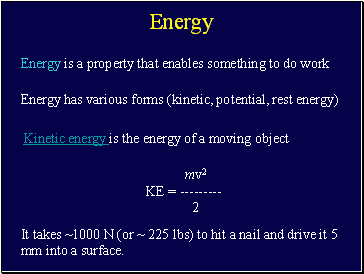## Energy

Energy is a property that enables something to do work

Energy has various forms (kinetic, potential, rest energy)

Kinetic energy is the energy of a moving object

mv2

KE = ---------

2

It takes ~1000 N (or ~ 225 lbs) to hit a nail and drive it 5 mm into a surface.

Slide 2## Potential Energy

Potential energy is a capacity of doing some work

Potential energy is the energy of position

Gravitational Potential Energy

W = Fd = mgh = PE (potential energy)

PE is relative!

PE of a 1000-kg car at the top of a 50-m multilevel parking lot is:

mgh= (1000 kg)(9.8 m/s2)(50 m)=490 kJ

Slide 3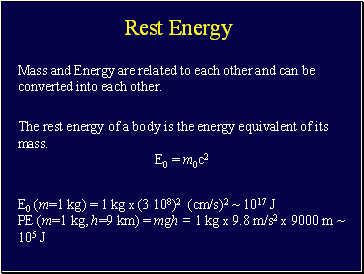## Rest Energy

Mass and Energy are related to each other and can be converted into each other.

The rest energy of a body is the energy equivalent of its mass.

E0 = m0c2

E0 (m=1 kg) = 1 kg x (3 108)2 (cm/s)2 ~ 1017 J

PE (m=1 kg, h=9 km) = mgh = 1 kg x 9.8 m/s2 x 9000 m ~ 105 J

Slide 4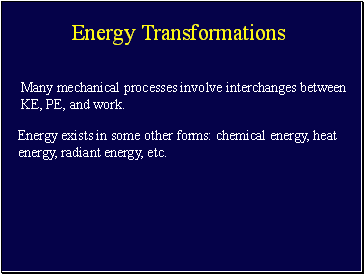## Energy Transformations

Many mechanical processes involve interchanges between KE, PE, and work.

Energy exists in some other forms: chemical energy, heat energy, radiant energy, etc.

Slide 5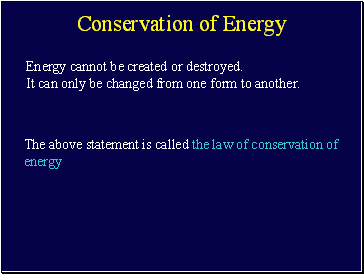## Conservation of Energy

Energy cannot be created or destroyed.

It can only be changed from one form to another.

The above statement is called the law of conservation of energy

Slide 6Chapter 5

Light and Atomic Structure

Light and its properties

Atomic structure

Interaction between Light and Matter

Spectrum

Slide 7## Light in Everyday Life

Light is a form of energy, radiative energy

1 Watt = 1 Joule/sec

Light has color

A prism split light into a spectrum (rainbow of colors)

Light travels with a speed of c = 300,000 km/s

Slide 8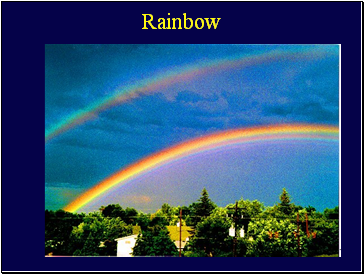Rainbow

Slide 9## Interaction of Light and Matter

Emission

Absorption

Transmission (passing through)

Reflection (scattering)

Slide 10## Properties of Light

Light behaves as both a particle and a wave

Light particles are called photons, which can be counted individually.

Go to page:
1  2  3  4